# Klein-Gordon equation

(Redirected from Klein–Gordon equation)

The relativistically-invariant quantum equation describing spinless scalar or pseudo-scalar particles, for example,-, and-mesons. The equation was established by O. Klein  and somewhat later by V.A. Fock [V.A. Fok] as a wave equation under the conditions of cyclicity in the fifth coordinate and was shortly afterwards deduced by several authors (for example, W. Gordon ) without this requirement on the fifth coordinate.

The subsequent application of the Klein–Gordon equation as a relativistic quantum equation proved possible in quantum field theory but not in quantum mechanics. In  an interpretation of the Klein–Gordon equation was given as an equation for fields of particles of zero spin. The Klein–Gordon equation is applied in the description of-mesons and corresponding fields; it plays the role of one of the fundamental equations of quantum field theory.

The Klein–Gordon equation is a linear homogeneous second-order partial differential equation with constant coefficients: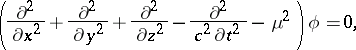(1)

where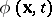is a (pseudo-) scalar function, in the general case — complex,andis the rest mass of the particle. Ifis a real function, then the Klein–Gordon equation describes neutral (pseudo-) scalar particles, while whenis complex it describes charged particles.

In the latter case equation (1) is supplemented by the equation for the complex-conjugate scalar function:(2)

The interaction of (pseudo-) scalar particles with the electromagnetic field is described by the minimal substitution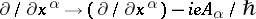. Each component of the wave function of particles of any spin also satisfies the Klein–Gordon equation, but only for the case where the spin is 0 is the function invariant with respect to the Lorentz–Poincaré group.

The Klein–Gordon equation can be obtained by means of the relationship between the energyand the momentum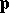of the particle in special relativity theory,by replacing quantities by operators (see , ):As for all relativistic equations, the Klein–Gordon equation can be expressed in the form of the Dirac equation, that is, it can be reduced to a first-order linear equation: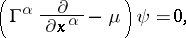(3)

where the coefficients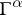are matrices similar to the Dirac matrices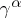. In the case of the Klein–Gordon equation the matricessatisfy the commutation relations: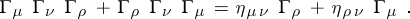(4)

For example,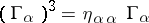(Kemmer–Duffin matrices). Hereis the metric tensor of Minkowski space. All theare singular matrices. Hence they do not have inverses.

Apart from the trivial solution,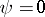to (4) and a solution in the form of five-row matrices, describing the scalar fielditself and the four components of its gradient, equation (4) has a further solution in the form of ten-row matrices. The corresponding ten-component function contains the four components of the potential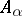and the six components of the stress, that is, equations (3) and (4) can simultaneously give a representation for the Proca equation describing vector particles with spin 1; for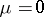and realthey give a representation of the Maxwell equations.

When taking into account the interaction of the (pseudo-) scalar particles with a gravity field in accordance with the general theory of relativity, the Klein–Gordon equation is generalized onto an arbitrary Riemannian space as: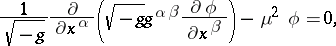(5)

whereis the metric tensor andis the determinant of the matrix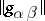. In equation (5) the termis frequently added, whereis the scalar curvature, as a result of which, when, the general relativistic Klein–Gordon equation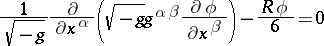becomes conformally invariant.